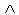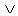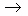Logic
edHelper subscribers - Create a new printable

Number of Keys
 Select the number of different printables: 1 key 2 keys 3 keys 4 keys 5 keys

Number of Pages (for each key)
 Select the number of pages: 1 page 2 pages 3 pages 4 pages

Include an answer key (answer keys will be at the end of the printable)
High School Geometry
High School Geometry
Math

 Name _____________________________Date ___________________
Logic
State the truth value of the following statements.

 1 If ~ p~ q is false, what conclusion can be drawn from the statement:~ pq
 2 If ~ pq is true, what conclusion can be drawn from the statement:( pq )( pq )
 3 If ~ p~ q is false, what conclusion can be drawn from the statement: q(~ pq )
 4 If p~ q is true, what conclusion can be drawn from the statement: pq
 5 If p~ q is true, what conclusion can be drawn from the statement:~(( p~ q )(~ p~ q ))
 6 If p~ q is true, what conclusion can be drawn from the statement:~( pq )
 7 If pq is false, what conclusion can be drawn from the statement:~( p~ q )~(~ qp )
 8 If pq is true, what conclusion can be drawn from the statement:(~ qp )(~ p~ q )
 9 If ~ pq is true, what conclusion can be drawn from the statement:~( p~ q )~(~ p~ q )
 10 If ~ pq is true, what conclusion can be drawn from the statement:~(~( p~ q )( q~ p ))
 11 If ~ pq is true, what conclusion can be drawn from the statement:(~ qp )~( q~ p )
 12 If ~ p~ q is true, what conclusion can be drawn from the statement:~ p~ q
 13 If p~ q is true, what conclusion can be drawn from the statement: pq
 14 If p~ q is false, what conclusion can be drawn from the statement:~(~( pq )~(~ qp ))
 15 If pq is false, what conclusion can be drawn from the statement: p~ q
 16 If ~ pq is false, what conclusion can be drawn from the statement:(~ pq )(~ q~ p )

Sample
This is only a sample worksheet.

Logic
edHelper subscribers - Create a new printable

Number of Keys
 Select the number of different printables: 1 key 2 keys 3 keys 4 keys 5 keys

Number of Pages (for each key)
 Select the number of pages: 1 page 2 pages 3 pages 4 pages